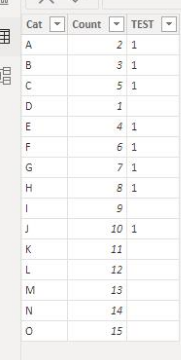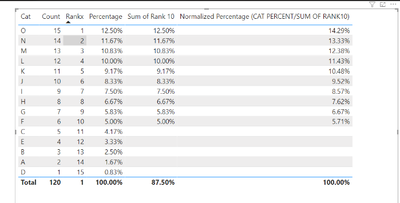cancel
Showing results for
Did you mean:Frequent Visitor

## Sum of Rank 10 Categories only

I have a table with categories and percentage for which i have calculated rank. I wanted to know what will be the sum of categories till Rank 10 only and i need to divide each percentage by sum of Rank10. Can anyone help?

 Cat Rank Percent Sum of Rank 10 Normalized Percentage (CAT PERCENT/SUM OF RANK10) A 1 11.76% 11.76% 18.05% B 2 10.60% 10.60% 16.27% C 3 7.11% 7.11% 10.91% D 4 6.24% 6.24% 9.58% E 5 5.95% 5.95% 9.13% F 6 5.66% 5.66% 8.69% G 7 4.93% 4.93% 7.57% H 8 4.79% 4.79% 7.35% I 9 4.35% 4.35% 6.68% J 10 3.77% 3.77% 5.79% K 11 3.39% L 12 3.03% M 13 4.31% N 14 2.88% O 15 8.38% Total 87.15% 65.16% 100%
1 ACCEPTED SOLUTIONCommunity Support

Hi , @MandoPavs008

Thanks for your quick response and sorry for the delay response due to the two days holiday.

According to your description, the "rankx" and the "Percentage" are all measures in your side.

Here are the steps you can refer to :

(1)This is my test data:(2)I create two measures like you to test:

Rankx = RANKX( ALL('Table'[Cat]) , CALCULATE( SUM('Table'[Count])))

Percentage = DIVIDE( SUM('Table'[Count]) , CALCULATE( SUM('Table'[Count]) ,ALL('Table')))

(3)Then we can create two measures:

Sum of Rank 10 = var _t =ADDCOLUMNS( ALLSELECTED('Table'[Cat]) , "rankx" , [Rankx],"Percentage",[Percentage])

var _cur_cat = VALUES('Table'[Cat])

var _t2 = FILTER( _t ,[rankx]<=10 && [Cat] in _cur_cat)

return

SUMX(_t2 , [Percentage])

Normalized Percentage (CAT PERCENT/SUM OF RANK10) = var _total_percentage= CALCULATE( [Sum of Rank 10] , ALLSELECTED('Table'[Cat]))

return

[Sum of Rank 10] / _total_percentage

(4)Then we can put the measures on the visual and we can meet your need , the result is as follows:Thank you for your time and sharing, and thank you for your support and understanding of PowerBI!

Best Regards,

Aniya Zhang

If this post helps, then please consider Accept it as the solution to help the other members find it more quickly

5 REPLIES 5Community Support

Hi , @MandoPavs008

Thanks for your quick response and sorry for the delay response due to the two days holiday.

According to your description, the "rankx" and the "Percentage" are all measures in your side.

Here are the steps you can refer to :

(1)This is my test data:(2)I create two measures like you to test:

Rankx = RANKX( ALL('Table'[Cat]) , CALCULATE( SUM('Table'[Count])))

Percentage = DIVIDE( SUM('Table'[Count]) , CALCULATE( SUM('Table'[Count]) ,ALL('Table')))

(3)Then we can create two measures:

Sum of Rank 10 = var _t =ADDCOLUMNS( ALLSELECTED('Table'[Cat]) , "rankx" , [Rankx],"Percentage",[Percentage])

var _cur_cat = VALUES('Table'[Cat])

var _t2 = FILTER( _t ,[rankx]<=10 && [Cat] in _cur_cat)

return

SUMX(_t2 , [Percentage])

Normalized Percentage (CAT PERCENT/SUM OF RANK10) = var _total_percentage= CALCULATE( [Sum of Rank 10] , ALLSELECTED('Table'[Cat]))

return

[Sum of Rank 10] / _total_percentage

(4)Then we can put the measures on the visual and we can meet your need , the result is as follows:Thank you for your time and sharing, and thank you for your support and understanding of PowerBI!

Best Regards,

Aniya Zhang

If this post helps, then please consider Accept it as the solution to help the other members find it more quicklyCommunity Support

Hi , @MandoPavs008

Based on your detailed requirement description and expected output data result provided, we can learn that you wanted to know what will be the sum of categories till Rank 10 only and you need to divide each percentage by sum of Rank10, I've made some local test and I figured a method that you can achieve this using two measures creating, here's my method, you can try:

Sum of Rank 10 =

var _value=IF(MAX('Table'[Rank])<=10,SUM('Table'[Percent]),BLANK())

var _total=SUMX(FILTER(ALLSELECTED('Table'),'Table'[Rank]<=10),'Table'[Percent])

return

IF(HASONEVALUE('Table'[Cat]),_value,_total)

Normalized Percentage (CAT PERCENT/SUM OF RANK10) =

var _total=SUMX(FILTER(ALLSELECTED('Table'),'Table'[Rank]<=10),'Table'[Percent])

return

IF(HASONEVALUE('Table'[Cat]),IF(MAX('Table'[Rank])<=10,DIVIDE(SUM('Table'[Percent]),_total),BLANK()),1)

And you can create a table visual to achieve this requirement like this:Thank you for your time and sharing, and thank you for your support and understanding of PowerBI!

Best Regards,

Aniya Zhang

If this post helps, then please consider Accept it as the solution to help the other members find it more quicklyFrequent Visitor

I tried with the measures i have but i got 100% for all under rank 10 in normalized percentage. I am unable to get the

 18.05% 16.27% 10.91% 9.58% 9.13% 8.69% 7.57% 7.35% 6.68% 5.79%Frequent Visitor

Thank you for the solution. Just wanted to update my question that rank and percenatge are measures and the actual table has only CAT and COUNT from which i have calculated measures for rank and percentage. So can you help me with how do i calculate sum of categories till Rank 10 only and divide percentage by sum of Rank10. I am kind of confused with using measures to calculate sum of categories till rank 10Impactful Individual

You can use the SUMX and FILTER functions in DAX to calculate the sum of the categories for the top 10 ranks, and then divide each percentage by that sum. Here is an example of how you might write the DAX code for this calculation:

SumRank10Categories:= SUMX(FILTER(Table,Table[Rank]<=10),Table[Category])

This measure will return the sum of the categories for the top 10 ranks.

To divide each percentage by the sum of top 10 categories, you can create another measure and divide it by the above measure.

DivideBySumRank10:=Table[Percentage]/[SumRank10Categories]

This measure will divide the percentage of each row by the sum of the top 10 categories.

You can then add this measure to your visual to see the result.

You can also use the measure in a calculated column to get the value for each row of your table.